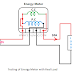The term "Phantom" means someone who appears to be present but really there is no one. In other words Phantom means ghosts.
So in case of energy meter, phantom load means some load is connected to the energy meter but actually there is no load. Phantom Loading is used for testing of energy meters.

To perform accurate testing we need to connect rated load to the energy meter.
During testing of energy meter, a virtual load is connected to the energy meter which is called phantom load.

Let's go to know how phantom load is provided to energy meter during testing.

Energy Meter has two coils one is Pressure Coil(P.C) or voltage coil and another one is the current coil(C.C). In the phantom loading technique, those two coils are excited separately.

The pressure coil(P.C) is excited by a voltage equal to the load voltage or rater voltage of that coil. Pressure coil has very high resistance, so there is no problem if the full rated voltage is given.

Current Coil(C.C) has very low resistance, so it is excited by very low voltage for which rated current will flow through the coil.

Now You may understand, there is no load connected to the energy meter but voltages are applied to those coils in such a way that it experiences the load is connected.

Now, you may think that why we did not use a real load.

It is very difficult to find the load which can take exact rated current and voltage.
Another problem is, if we used real load it will waste huge power.

Let's go to understand with an example.

Suppose a DC energy meter having a rating of 230V and 10A. The resistance of the pressure coil and the current coil is 5290 ohm and 0.1 Ohm respectively.

Now if we connect a real load which takes 10A current at 230V.

The power consumption in pressure coil will be {(230)^2}/5290 = 10 watt
The power consumption in the current coil will be 230*9 = 2070 watt
So the total power consumption is 10+2070 = 2080 watt.

[Explained] Phantom Loading in Energy MeterReviewed by manoranjan das on 11:36 am Rating: 5